##### Trigonometry Workbook For DummiesYou don’t see many telescoping series, but the telescoping series rule is a good one to keep in your bag of tricks — you never know when it might come in handy. Consider the following series: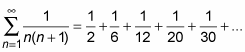To see that this is a telescoping series, you have to use the partial fractions technique to rewrite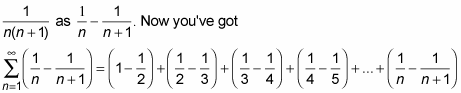All these terms now collapse, or telescope. The 1/2s cancel, the 1/3s cancel, the 1/4s cancel, and so on. All that’s left is the first term, 1 (actually, it’s only half a term), and the last half-term,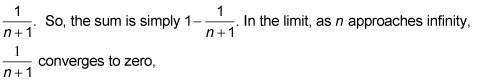and thus the sum converges to 1 – 0, or 1.

You can write each term in a telescoping series as the difference of two half-terms — call them h-terms. You can then write the telescoping series as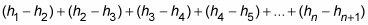Here's the telescoping series rule: A telescoping series of the above form converges if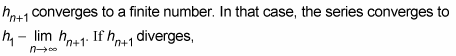then the series diverges.

This rule, like the rule for geometric series, lets you determine what number a convergent telescoping series converges to.